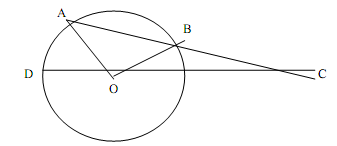In the figure given below, AB is the chord of a circle with center O. AB is extended to C such that

BC = OB. The straight line CO is produced to meet the circle at D. If  ? ACD = y degrees and ? AOD = x degrees such that x = ky, then the value of k is